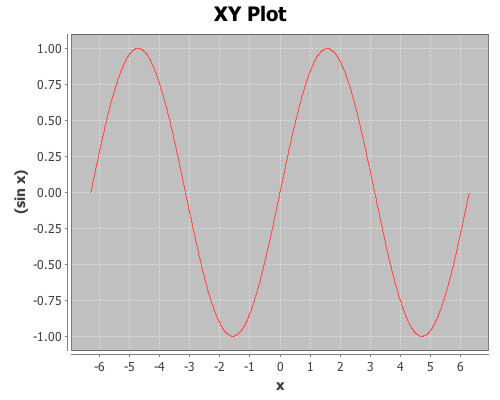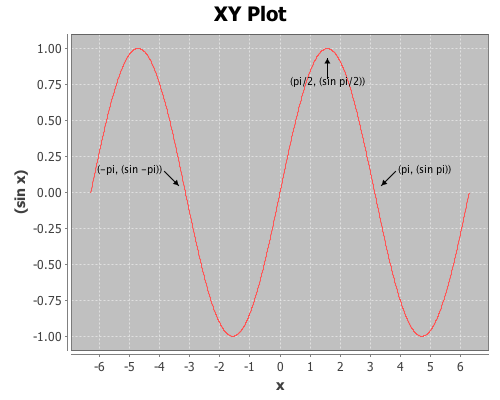# Annotating Incanter plots

The following examples will demonstrate how to annotate Incanter plots using the add-pointer, add-text, and add-polygon functions. You will need the incanter.core, incanter.stats, incanter.charts, and incanter.datasets libraries. For more information on using these libraries, see the matrices, datasets, and sample plots pages on the Incanter wiki.

``(use '(incanter core stats charts datasets))``

and plotting the sin function using the function-plot function.

``````(def plot (function-plot sin (* -2 Math/PI) (* 2 Math/PI)))
(view plot)``````Now annotate a few points on the plot with the add-pointer function.

``````(doto plot
(add-pointer (- Math/PI) (sin (- Math/PI))
:text "(-pi, (sin -pi))")
:text "(pi, (sin pi))" :angle :ne)
(add-pointer (* 1/2 Math/PI) (sin (* 1/2 Math/PI))
:text "(pi/2, (sin pi/2))" :angle :south))``````add-pointer’s :angle option changes the direction the arrow is pointing. A number representing the angle, or a keyword representing a direction can be passed as arguments.

Here’s an example of each of the directions.

``````(doto plot
(add-pointer 0 0 :text "north" :angle :north)
(add-pointer 0 0 :text "nw" :angle :nw)
(add-pointer 0 0 :text "ne" :angle :ne)
(add-pointer 0 0 :text "west" :angle :west)
(add-pointer 0 0 :text "east" :angle :east)
(add-pointer 0 0 :text "south" :angle :south)
(add-pointer 0 0 :text "sw" :angle :sw)
(add-pointer 0 0 :text "se" :angle :se))``````This next example will demonstrate using the add-text and add-polygon functions by annotating the iris PCA scatter-plot generated in an earlier post. The code for creating this plot can be found here.Now add the species names to each cluster.

``````(doto plotThe text is centered at the given coordinates. Finally place a box around the Setosa group.

``` ```
``````(add-polygon plot
[[-3.2 -6.3] [-2 -6.3] [-2 -3.78] [-3.2 -3.78]])``````Shapes are not limited to rectangles, add as many coordinates as necessary to create arbitrary polygons; the last point will automatically connect to the first one. If only two coordinates are provided a single line is added to the plot.

Incanter charts are instances of the JFreeChart class from the JFreeChart library, so additional customizations can be achieved by using the underlying Java APIs directly.

The complete code for this example can be found here.